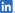Focal PointFocal Point ForumsWebFOCUS/FOCUS Forum on Focal Point[CLOSED] Mod10 Calculation
 GoNewSearchNotifyToolsReply[CLOSED] Mod10 CalculationSilver Memberposted January 23, 2013 04:00 PM
Has anyone built a compute or define for
calculating a Modulus 10 check digit on a variable length number? If so, I would be very grateful if you could share this with me?

This message has been edited. Last edited by: Kerry,

WEBFOCUS 7.14
WEBFOCUS.8.04

 Posts: 44 | Registered: March 30, 2007IP
Expertposted January 23, 2013 04:08 PM Hide Post
I think someone here did it, but did it by calling an OS command.

Waz...

 Prod: WebFOCUS 7.6.10/8.1.04 Upgrade: WebFOCUS 8.2.06 OS: Linux Outputs: HTML, PDF, Excel, PPT In Focus since 1984 Know The CodePosts: 6207 | Location: 33.8688° S, 151.2093° E | Registered: October 31, 2006IP
Virtuosoposted January 23, 2013 06:32 PM Hide Post
Take a look at the function IMOD
I think that will do what you need

 Frankprod: WF 7.6.10 platform Windows, databases: msSQL2000, msSQL2005, RMS, Oracle, Sybase,IE7test: WF 7.6.10 on the same platform and databases,IE7

 Posts: 2387 | Location: Amsterdam, the Netherlands | Registered: December 03, 2006IP
Virtuosoposted January 24, 2013 10:02 AM Hide Post
Cathy,
There are many different formulae for calculating a Modulus 10 check digit.
For example:
You can add all the digits and find the remainder when dividing by 10.
You can have different multipliers for each digit.
You can take the complement for the check digit calculated in one of the above examples.
So, which is yours?

Daniel
In Focus since 1982
wf 8.202M/Win10/IIS/SSA - WrapApp Front End for WF

 Posts: 1941 | Location: Tel Aviv, Israel | Registered: March 23, 2006IP
Masterposted January 26, 2013 05:27 PM Hide Post
quote:
...calculating a Modulus 10 check digit on a variable length number...

The following focexec...
```-* File TheModSquad.fex
APP PREPENDPATH IBISAMP
-*
DEFINE FUNCTION GET_DIGIT(SN/D20, X1/D20)
GET_DIGIT/I2 = DMOD(SN, X1, 'D2') / (X1 / 10);
END
-*
DEFINE FUNCTION SUM_DIGIT(DIVIDEND/I2)
SUM_DIGIT/I1 = (IMOD(DIVIDEND, 100, 'I2') / 10) + IMOD(DIVIDEND, 10, 'I2')  ;
END
-*
DEFINE FILE CAR
-* Generating numbers to use as test cases (starting numbers).
START_NUM/D10 = DECODE CAR ('DATSUN' 7992739871
'AUDI'   23
'TOYOTA' 5556);
-* Add a zero to the end of the Starting Number.
START_NUM_D20/D20 = START_NUM * 10;
-* Double every other digit, and then sum the resulting digit(s).
DIGIT02/I2 = SUM_DIGIT(GET_DIGIT(START_NUM_D20, 100) * 2);
DIGIT03/I2 = SUM_DIGIT(GET_DIGIT(START_NUM_D20, 1000));
DIGIT04/I2 = SUM_DIGIT(GET_DIGIT(START_NUM_D20, 10000) * 2);
DIGIT05/I2 = SUM_DIGIT(GET_DIGIT(START_NUM_D20, 100000));
DIGIT06/I2 = SUM_DIGIT(GET_DIGIT(START_NUM_D20, 1000000) * 2);
DIGIT07/I2 = SUM_DIGIT(GET_DIGIT(START_NUM_D20, 10000000));
DIGIT08/I2 = SUM_DIGIT(GET_DIGIT(START_NUM_D20, 100000000) * 2);
DIGIT09/I2 = SUM_DIGIT(GET_DIGIT(START_NUM_D20, 1000000000));
DIGIT10/I2 = SUM_DIGIT(GET_DIGIT(START_NUM_D20, 10000000000) * 2);
DIGIT11/I2 = SUM_DIGIT(GET_DIGIT(START_NUM_D20, 100000000000));
-* Add each, and multiply by 9.
CHECKIT/D3 = (DIGIT02 + DIGIT03 + DIGIT04 + DIGIT05 + DIGIT06 +
DIGIT07 + DIGIT08 + DIGIT09 + DIGIT10 + DIGIT11) * 9;
-* Pull the check digit.
CHECKDIGIT/D1 = DMOD(CHECKIT, 10, 'D1');
-* Create the final full output number.
FULL_NUMBER/D22c = START_NUM_D20 + CHECKDIGIT;
END
-*
TABLE FILE CAR
PRINT START_NUM   AS 'Starting/Input Number'
FULL_NUMBER AS 'Final Full/Output Number'
IF    CAR EQ 'DATSUN' OR 'AUDI' OR 'TOYOTA'
ON TABLE SET PAGE NOPAGE
ON TABLE SET ONLINE-FMT STANDARD
END```

Produces the following output..
```Starting/Input Number  Final Full/Output Number
---------------------  ------------------------
7,992,739,871               79927398713
5,556                     55566
23                       232
```

All tests pass the LUHN-10 Checking tool at http://www.ee.unb.ca/cgi-bin/tervo/luhn.pl

 Test: WebFOCUS 8.1.05M Prod: WebFOCUS 8.1.05M Server: Windows Server 2012/Tomcat Standalone Workstation: Windows 7/IE 11 Database: Oracle 12c/Netezza Output: AHTML/XLSX/HTML/PDF/JSCHART Tools: WFDS, Repository Content, BI Portal Designer, & ReportCasterPosts: 786 | Registered: April 23, 2003IP
Platinum Memberposted January 28, 2013 12:00 AM Hide Post
Here's a pure Dialogue Manager solution I wrote.
&n = the number you are starting with.
&c = is the check digit calculated from &n.
```
-DEFAULT &n = '7992739871';
-SET &c = 0;
-SET &o = IMOD(&n.LENGTH + 1, 2, 'I1');
-REPEAT ENDREPEAT FOR &i FROM &n.LENGTH TO 1 STEP -1
-SET &d = SUBSTR(&n.LENGTH, &n, &i, &i, 1, 'A1');
-SET &c = IF IMOD(&i, 2, 'I1') EQ &o THEN (&c + &d) ELSE (&c + DECODE &d (0 0 1 2 2 4 3 6 4 8 5 1 6 3 7 5 8 7 9 9));
-ENDREPEAT
-SET &c = IMOD(&c * 9, 10, 'I1');
-TYPE Number = &n   Check Digit = &c
```

WF 8.2.06
Win10 / IE11
AHTML EXL2K PDF

 Posts: 147 | Registered: October 19, 2010IP
Silver Memberposted January 30, 2013 08:56 AM Hide Post
thanks so much I will give these examples a try and post back my results!

WEBFOCUS 7.14
WEBFOCUS.8.04

 Posts: 44 | Registered: March 30, 2007IP
 ReplyFocal PointFocal Point ForumsWebFOCUS/FOCUS Forum on Focal Point[CLOSED] Mod10 Calculation